summary of site-wide JavaScript functionalityUnited States-English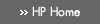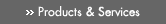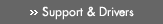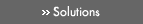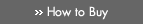» Contact HPSearch:More optionsManualTechnical documentation - EnglishAll of HP USHP-UX Reference > L# logb(3M)

HP-UX 11i Version 3: February 2007»

## Technical documentation» Feedback## » Index## NAME

logb(), logbf(), logbl(), logbw(), logbq() — radix-independent exponent functions

## SYNOPSIS

#include <math.h>

double logb(double x);

### HP Integrity Server Only

float logbf(float x);

long double logbl(long double x);

extended logbw(extended x);

## DESCRIPTION

The logb() function computes the exponent of the floating point value x. Formally, the return value is the integral part of log base r of |x| as a signed floating point value, for nonzero x, where r is the radix of the machine's floating-point arithmetic. The radix r is 2 on HP-UX systems.

If x is denormal, it is treated as though it were normalized, before the exponent is determined.

### Integrity Server Only

logbf() is a float version of logb(); it takes a float argument and returns a float result.

logbl() is a long double version of logb(); it takes a long double argument and returns a long double result.

logbw() is an extended version of logb(); it takes an extended argument and returns an extended result.

logbq() is equivalent to logbl() on HP-UX systems.

## USAGE

To use these functions, compile either with the default -Ae option or with the -Aa and -D_HPUX_SOURCE options.

To use (for Integrity servers) logbw() or logbq(), compile also with -fpwidetypes.

Make sure your program includes <math.h>. Link in the math library by specifying -lm on the compiler or linker command line. For more information, see the HP-UX floating-point guide for HP Integrity servers at the following site: http://www.hp.com/go/fp.

## RETURN VALUE

Upon successful completion, logb() returns the exponent of x.

If x is NaN, logb() returns NaN.

If x is ±INFINITY, logb() returns +INFINITY.

If x is zero, logb() returns -HUGE_VAL (equal to -INFINITY) and raises the divide-by-zero floating-point exception.

## ERRORS

If x is zero, logb() sets errno to [EDOM].

### Integrity Server Only

HP-UX libm functions on Integrity servers do not set errno by default. For errno setting, compile with the +Olibmerrno option.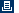Printable versionPrivacy statement Using this site means you accept its terms Feedback to webmaster© 1983-2007 Hewlett-Packard Development Company, L.P.